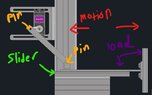# Required torque calculation

• mountainchicken46
In summary, the conversation is about how to calculate the required torque for a mini servo to move a load on a slider. The mechanism in question is a highly offset slider-crank mechanism. The purpose of the movement is to either hold the load against a constant velocity of 0.5m/s or accelerate the load at a specific rate. The discussion also touches on the limitations of the slider's range and the need to control the crank speed for varying the velocity.

#### mountainchicken46

TL;DR Summary
how to calculate the required torque for the mini servo to move the load on slider?
how to calculate the required torque for the mini servo to move the load on slider?

#### Attachments

•Screenshot (52)_LI.jpg
30.7 KB · Views: 111
Welcome to PhysicsForums.mountainchicken46 said:
Summary:: how to calculate the required torque for the mini servo to move the load on slider?

how to calculate the required torque for the mini servo to move the load on slider?
You go first...•anorlunda
What you have is a highly offset slider-crank mechanism.

Are you concerned with simply holding the slider against a load, or do you want to accelerate the slider? If you want to accelerate the slider, then at what rate? You need to give us a bit more to work with.

I am confused.
How many separate parts are there ?
What can move relative to what ?
What bits are the fixed frame or chassis ?

Dr.D said:
What you have is a highly offset slider-crank mechanism.

Are you concerned with simply holding the slider against a load, or do you want to accelerate the slider? If you want to accelerate the slider, then at what rate? You need to give us a bit more to work with.
I want to move the load (it is actually a heater) that was mounted on the slider at 0.5m/s either to the left or right.

A constant velocity on the velocity will require a variable crank speed. Are you prepared to control crank speed to vary in a specific way?

I hope you are aware that the slider moves only over a limited range. As you approach the ends of the range, the velocity must approach zero in order to reverse. Is this satisfactory for your purposes?

## 1. What is required torque calculation?

Required torque calculation refers to the process of determining the amount of rotational force, or torque, needed to move or hold a specific object or system.

## 2. Why is required torque calculation important?

Required torque calculation is important because it helps engineers and designers ensure that the proper amount of torque is applied to a system or object in order to achieve the desired motion or holding force. This is crucial for the safe and efficient operation of machinery and equipment.

## 3. How is required torque calculated?

The required torque can be calculated by using the formula T = F x r, where T is torque, F is the force applied to the system, and r is the distance from the point of rotation to the force. This formula can be used for both linear and rotational motion, but may vary depending on the specific system or object being analyzed.

## 4. What factors affect required torque?

The amount of required torque can be influenced by several factors such as the weight and size of the object, friction, and the type of motion or force being applied. Other factors may include the speed of rotation and the material properties of the system.

## 5. How can required torque be optimized?

Required torque can be optimized by reducing friction, using the appropriate lubricants, and designing the system with efficient and precise components. Additionally, regularly maintaining and calibrating the system can ensure that the required torque remains at optimal levels.